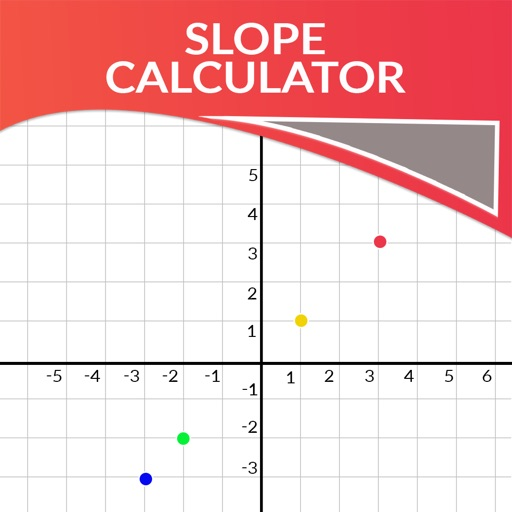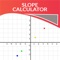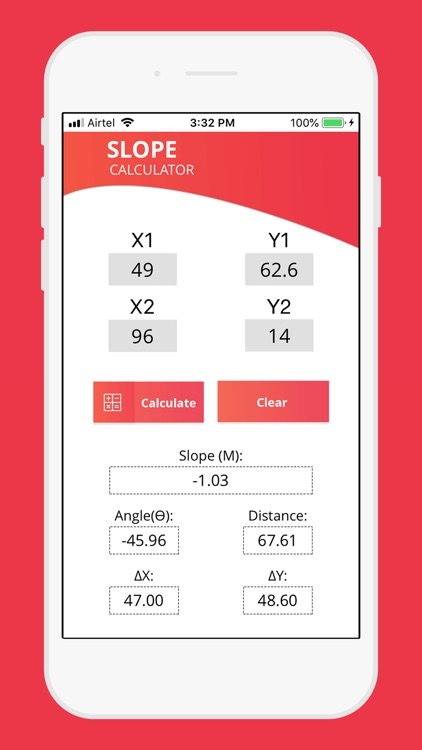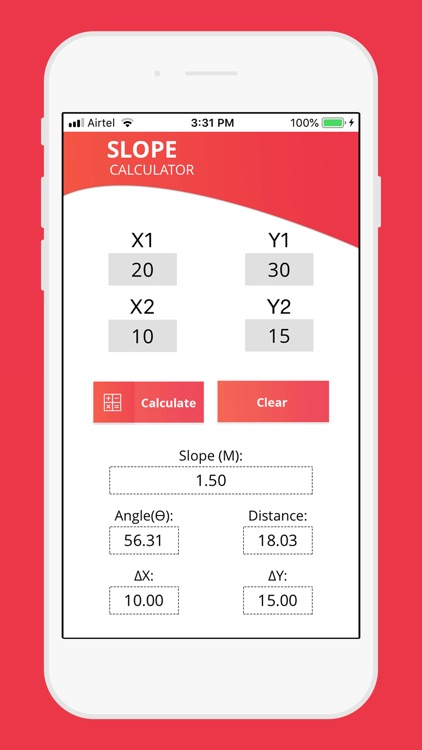## The slope calculator determines the slope or gradient between two points in the Cartesian coordinate system# Slope Calculator+

by Gorasiya Vishal NanjibhaiThe slope calculator determines the slope or gradient between two points in the Cartesian coordinate system. The slope is basically the amount of slant a line has and can take on positive, negative, zero or an undefined value. Before we can use the calculator we might want to learn how to find the slope using the slope formula.Version
1.0
Rating
(2)
Size
1Mb
Genre
Utilities
Last updated
December 8, 2018
Release date
December 7, 2018